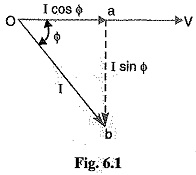## Power Factor:

The electrical energy is almost exclusively generated, transmitted and distributed in the form of alternating current. Therefore, the question of power factor immediately comes into picture. Most of the loads (e.g. induction motors, arc lamps) are inductive in nature and hence have low lagging power factor. The low power factor is highly undesirable as it causes an increase in current, resulting in additional losses of active power in all the elements of power system from power station generator down to the utilization devices. In order to ensure most favorable conditions for a supply system from engineering and economical standpoint, it is important to have power factors as close to unity as possible. In this category, we shall discuss the various methods of power factors improvement.

### Power Factor Definition:

The cosine of angle between voltage and current in an a.c. circuit is known as power factor.

In an a.c. circuit, there is generally a phase difference Φ between voltage and current. The term cos Φ is called the power factors of the circuit. If the circuit is inductive, the current lags behind the voltage and the power factor is referred to as lagging. However, in a capacitive circuit, current leads the voltage and power factors is said to be leading.Consider an inductive circuit taking a lagging current I from supply voltage V; the angle of lag being Φ. The phasor diagram of the circuit is shown in Fig. 6.1. The circuit current I can be resolved into two perpendicular components, namely ;

• I cos Φ in phase with V
• I sin Φ 90° out of phase with V

The component I cos Φ is known as active or wattful component, whereas component I sin Φ is called the reactive or wattless component. The reactive component is a measure of the power factor. If the reactive component is small, the phase angle Φ is small and hence power factor cos Φ will be high. Therefore, a circuit having small reactive current (i.e., I sin Φ) will have high power factor and vice-versa. It may be noted that value of power factors can never be more than unity.

• It is a usual practice to attach the word ‘lagging’ or ‘leading’ with the numerical value of power factors to signify whether the current lags or leads the voltage. Thus if the circuit has a p.f. of 0.5 and the current lags the voltage, we generally write p.1 as 0.5 lagging.
• Sometimes power factors is expressed as a percentage. Thus 0.8 lagging power factor may be expressed as 80% lagging.
Scroll to Top